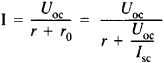# Open-Circuit and Short-Circuit Method

The following article is from The Great Soviet Encyclopedia (1979). It might be outdated or ideologically biased.

## Open-Circuit and Short-Circuit Method

(known in English as Thévenin’s theorem), a theorem that provides for the calculation or experimental determination of operating conditions in a branch of a linear electrical network or in a linear electrical device, such as an electric machine, a battery, or an amplifier. The simplest mathematical relationships are obtained for DC networks. However, the theorem may also be applied to AC networks, both with sinusoidal currents and with nonsinusoidal currents; it may also be used to investigate transient processes.

The essence of Thévenin’s theorem consists in representing a whole network as two separate components, namely, an active two-terminal network and the branch under investigation, which has an impedance r and is connected to the active two-terminal network. In the absence of a load (that is, when the branch with impedance r is disconnected), the two-terminal network is opencircuited. The voltage between the two terminals is called the open-circuit voltage Uoc. This voltage may be calculated or may be measured with a voltmeter having a sufficiently high (in theory, an infinitely high) impedance. When the two terminals are connected by a conductor or by means of an ammeter with a sufficiently low impedance (in theory, an impedance of zero), the two-terminal network is short-circuited, and a short-circuit current Isc, which may be calculated or measured, flows through the conductor or across the ammeter. The ratio Uoc/Isc is equal to the internal, or input, impedance r0 of the active two-terminal network. Under operating conditions, the current I flowing through the branch with impedance r is given by the expressionIn theoretical works, Thévenin’s theorem is also called Helmholtz’s theorem; when admittances are used, it is called Norton’s theorem.

### REFERENCES

Lineinye elektricherskie tsepi s sosredotochennymi postoiannymi. Moscow, 1972. (Teoreticheskie osnovy elektrotekhniki, vol. 1.)
Osnovy teorii Isepei, 4th ed. Moscow, 1975.

B. IA. ZHUKHOVITSKII